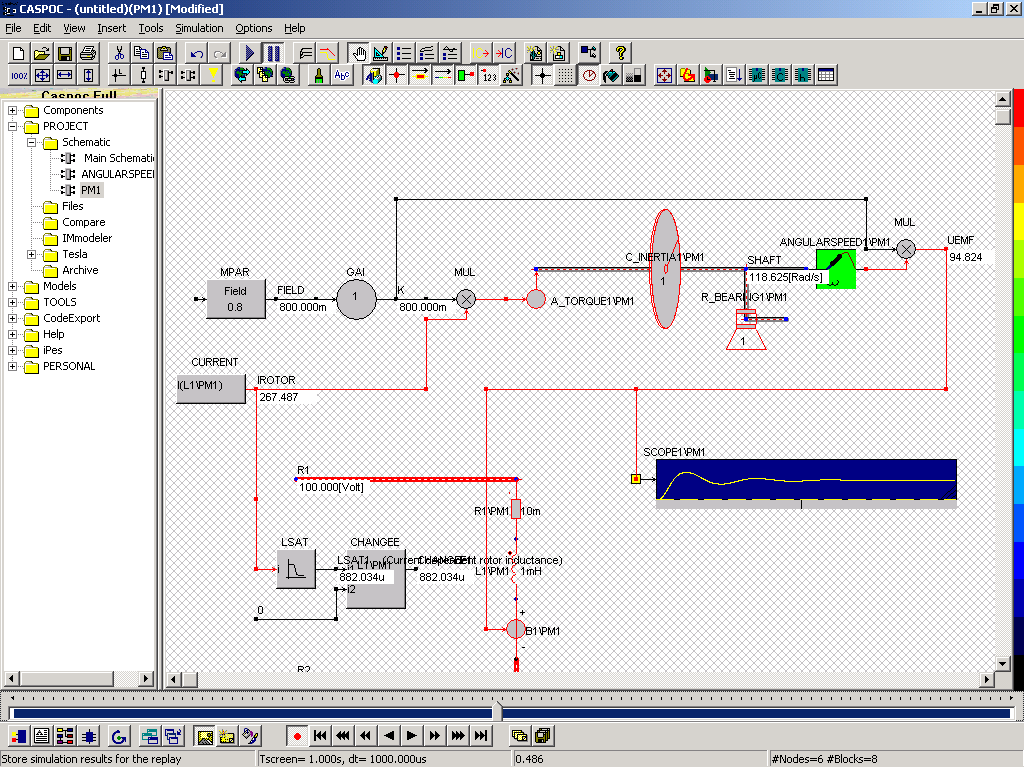• Introduction
• What is in this manual
• What is Caspoc
• User interface
• Introduction
• Starting
• Simulation
• Editing
• Viewing and printing
• Getting Started
• Basic editing
• Simulation in the time domain
• Basic User Interface Topics
• Editing
• Simulation
• Viewing
• Library
• Reports
• Project management
• Circuit and Block Diagram Components
• Introduction
• Cscript and user defined functions
• Component parameters
• Modeling Topics
• Introduction
• Power Electronics
• Semiconductors
• Electrical Machines
• Electrical drives
• Power Systems
• Mechanical Systems
• Thermal Systems
• Magnetic Circuits
• Green Energy
• Coupling to FEM
• Experimenter
• Analog hardware description language
• Embedded C code Export
• Coupling to Spice
• Small Signal Analysis
• Matlab coupling
• Tips and tricks
• Appendices

## Create electrical machine models

In this tutorial we will create machine models from scratch. This means that you will learn how to set up machine models and how to modify existing machine models.

First we will discuss the basics of the model, the electrical and mechanical connections. This will be done using a simple DC machine example, since these are simple equations. First a circuit model is created with linear components. This model will be extended with non-linear components, for example saturation. The next step is to model everything using block diagram components and controlled sources.

Our final example is a BLDCM where a combination of a circuit model and a block diagram model is created. This model is first created with linear components. Next, non-linear components are introduced and finally results from a FEM analysis are used to model the back-emf of the BLDCM.

Further topics that will be discussed are; reading parameters from a .model database, multiplexing variables into one sensor connection, display of phasors

Basics of a machine model

Each machine model has electrical connections and a mechanical shaft. If we create a machine model we also define terminals in our model where we can supply parameters and a sensor terminal that can be used to read all currents, voltages, flux and other variables in our model.

But lets first have a look at the basic mechanical system.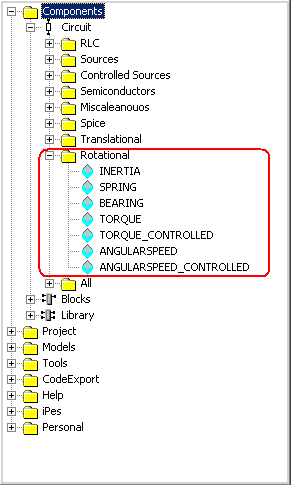By selecting Components/Circuit/Rotational from the Project Manager you see the overview of the mechanical components in Caspoc. Since we want to create a rotating machine, we select the rotational components. In our machine model we would like to have a mechanical shaft. We would like to calculate the torque that is produced in the electrical machine and use that torque for rotating the shaft. Therefore we select the controlled torque source and place it on our workscreen

(Select the component by clicking and releasing it with the left mouse button in the Project Manager, on the workscreen, click and release the left mouse button to place the component)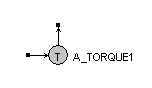If we draw wires connected to the controlled source, you will notice that here are two types of wires. The wire on the left side is the numerical value of the torque that is used to control the controlled source. You will see that the node is square. The wire on the right side is the actual mechanical shaft of the machine. The shaft is drawn with a thicker line (if the animation is enabled and the line thickness is specified in the animation properties).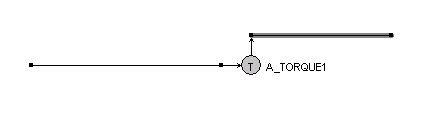The next step is to create some mechanical load for the machine and also to specify the torque of the machine.

The rotor of the machine is dominantly defined by the inertia of the rotor. The inertia is the rotating mass of the machine and is modeled by the component Inertia and its value is in [Kgm2]. For simplicity we choose 1 Kgm2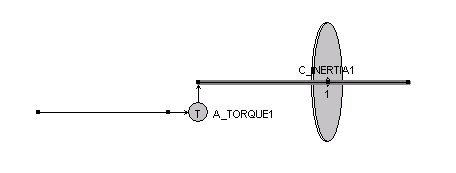Suppose we would have some sort of permanent magnet motor. We assume that the stator contains permanent magnets and that the rotor is fed from a commutator. The torque of the machine is mostly the product of field, a motor constant K and current. Therefore we add a multiplier where the inputs are the field (from permanent magnets) and the current (from the windings) are multiplied. Suppose that the field has a constant distribution among the windings and that it has a value of 1 Tesla. The current through the windings will be measured and used in the multiplier.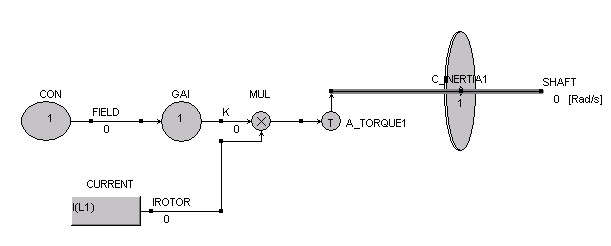Our mechanical model is ready and the next step is the electrical part. We assume that an inductor can model the electrical windings in the machine. We also add some DC winding resistance in series with the inductor to model the copper losses in the windings.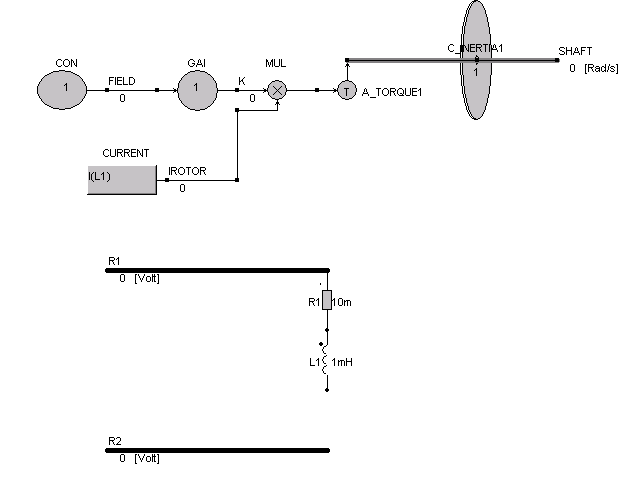In the above figure here is still some space left open in the electric circuit. This is where the back-emf has to be modeled. Because the electrical input power of the machine has to be nearly equal to the mechanical output power of the machine minus the losses, the back emf can be calculated from:

Pin= u*I ~ Uemf * iL1

Pout= T * omega

Uemf=T * omega / iL1 = K* Field * IL1 * omega / iL1 = K * Field * omega

A controlled voltage source, a multiplier and an angular speed sensor model the back-emf.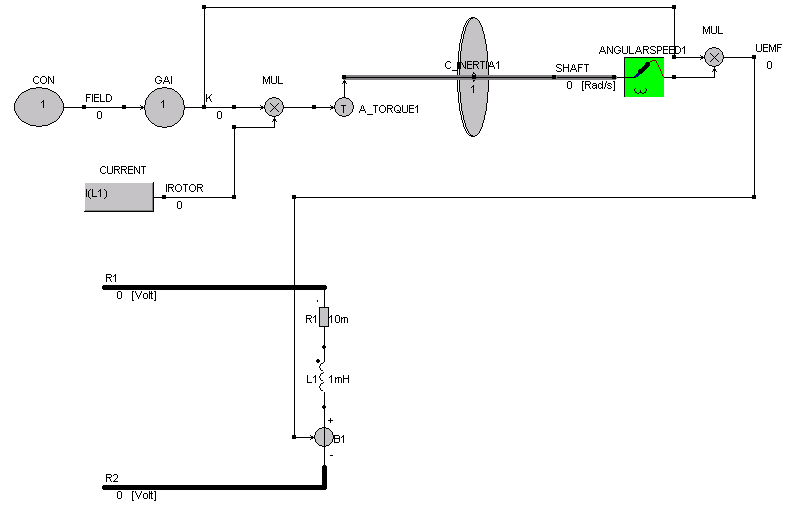Our model is ready for the first try. We add a voltage source to drive the machine and add a scope to see the speed of the machine. To have some load for the machine, we add a bearing to the shaft. The voltage source connected to the machine has a value of 100volt. The label R1 is changed into 0 to ground the electrical part of the circuit.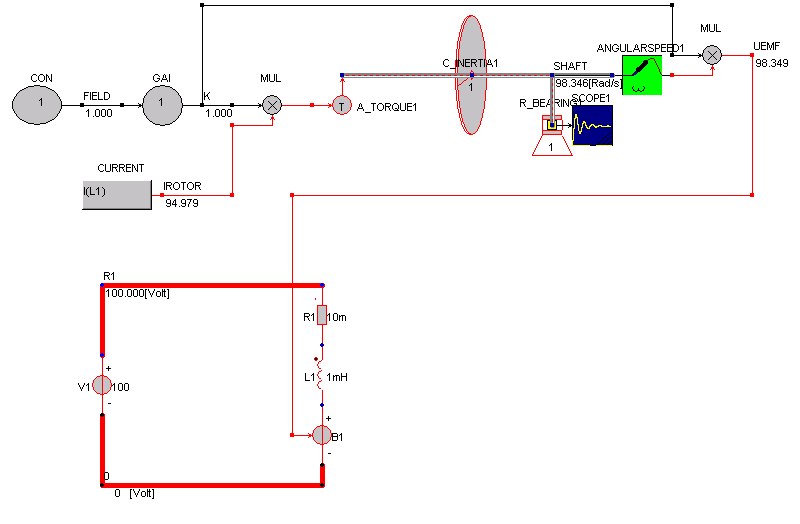The parameters for the simulation are specified in the dialog box simulation parameters. Here we specify a total simulation time of 1 second and a time step of 1ms.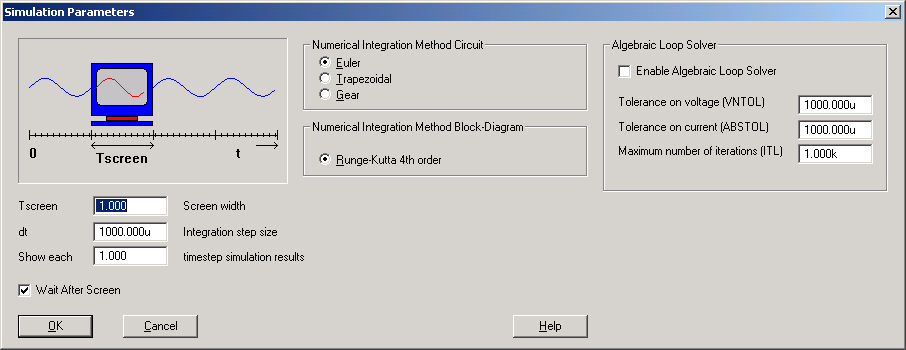We can open the scope to see how the start up of the machine is simulated.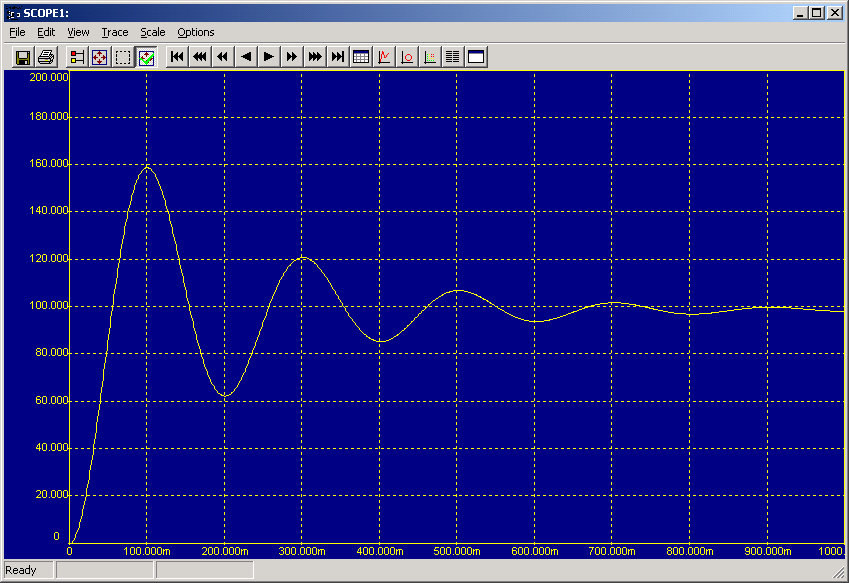We are also interested in the current during start up. Therefore we extend the scope and connect the second input of the scope to the inductor.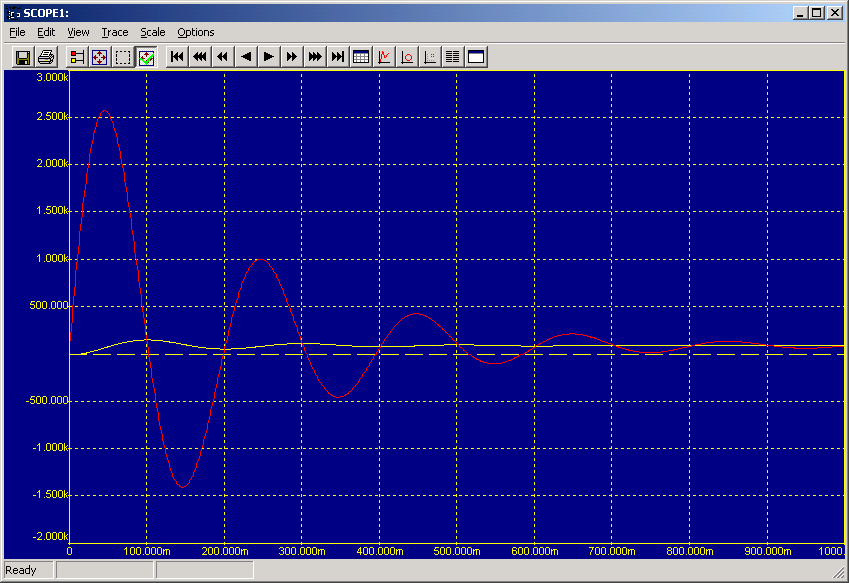As can be seen from this scope, the starting current is rather high and there are oscillations in the angular speed and current.

To use this model as one block, we will have to export it into a library block. We have to define which labels nodes have to be exported. These are the shaft, and the two electrical nodes. Select the nodes with the right mouse button and select the export direction.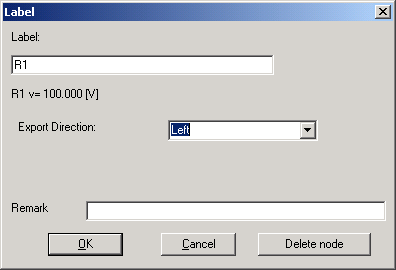The exported labels are identified in the schematic by a little flag pointing in the export direction.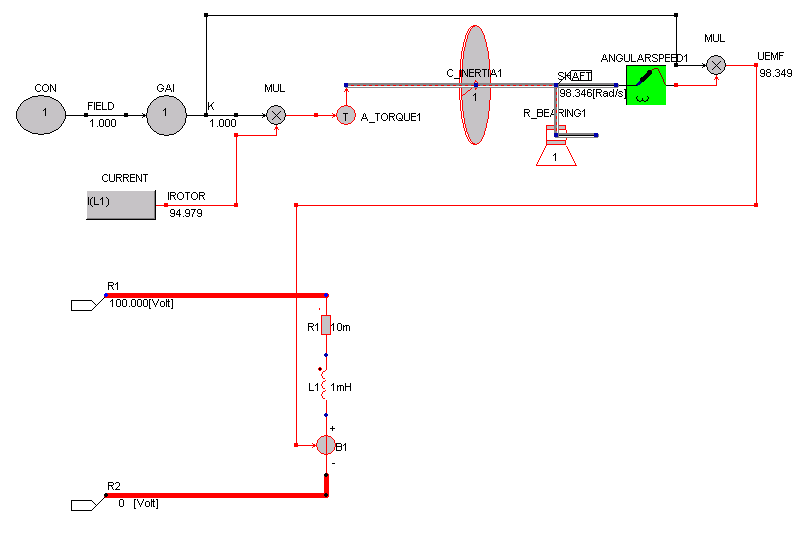Suppose that the field should be an external parameter that has to be specified by the user, we will replace the block CON by a MPAR block. If the model is exported into one library block, the user can enter the value of the field in the properties dialog box of the exported machine model.In the properties dialog box for the MPAR block specify the name of the label and its default value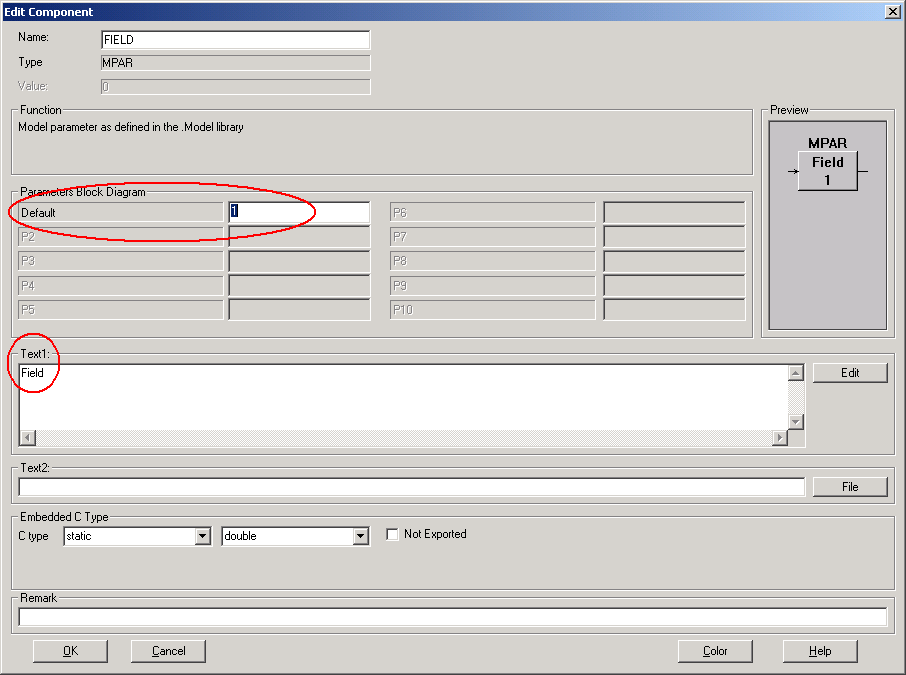If all nodes that have to be exported are defined and a MPAR block replaces the CON block, we are ready to export the block. From the menu select Edit/Export Library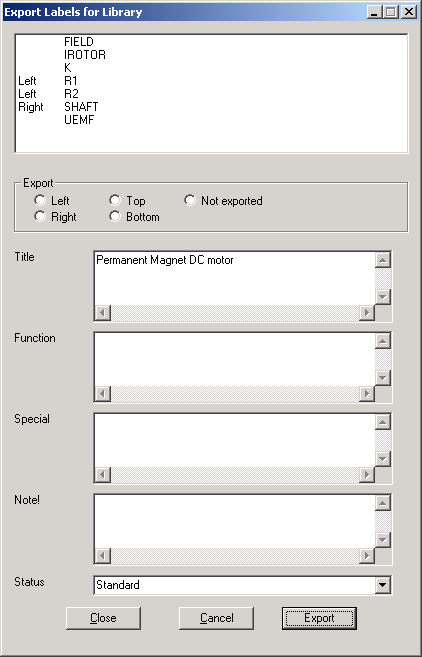and select the button [Export] and save it under the name of MyPermanentMagnetMotorThe model is stored in the file MyPermanentMagnetMotor.lib and is ready for multiple use in a schematic.

Start with anew schematic by selecting File/New and import the PM motor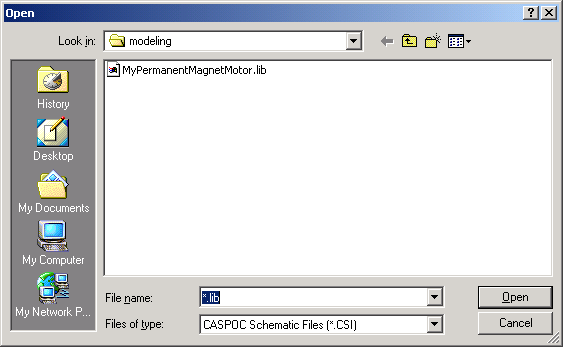During the import of the library block, you can specify its namePlace the PM motor on your schematic. Since we have no icon defined, we will see only the gray block and the labels.Lets first connect the block to a voltage source and see if the machine is running.We also defined the node ground, which is required in circuit simulation. If we run the simulation, the angular speed is shown in the scope.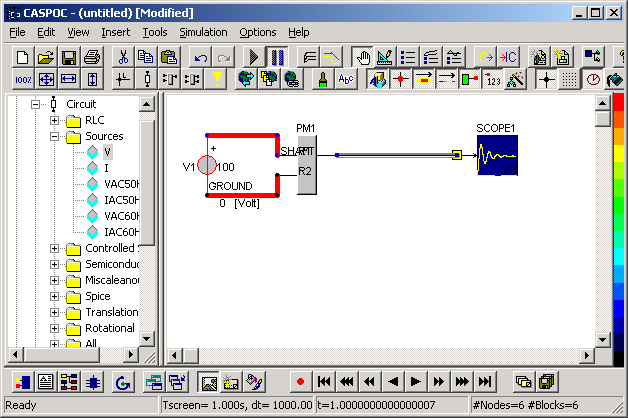Opening the scope shows that our angualr speed reaches nearly 100 Rad/s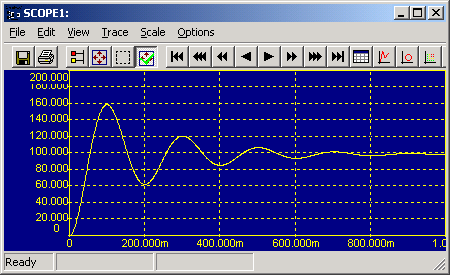Our parameter Field is now accessable by the user, from the proprties dialog box. Open the properties dialog box with the right mouse buton and change the value of the field into 2 Tesla.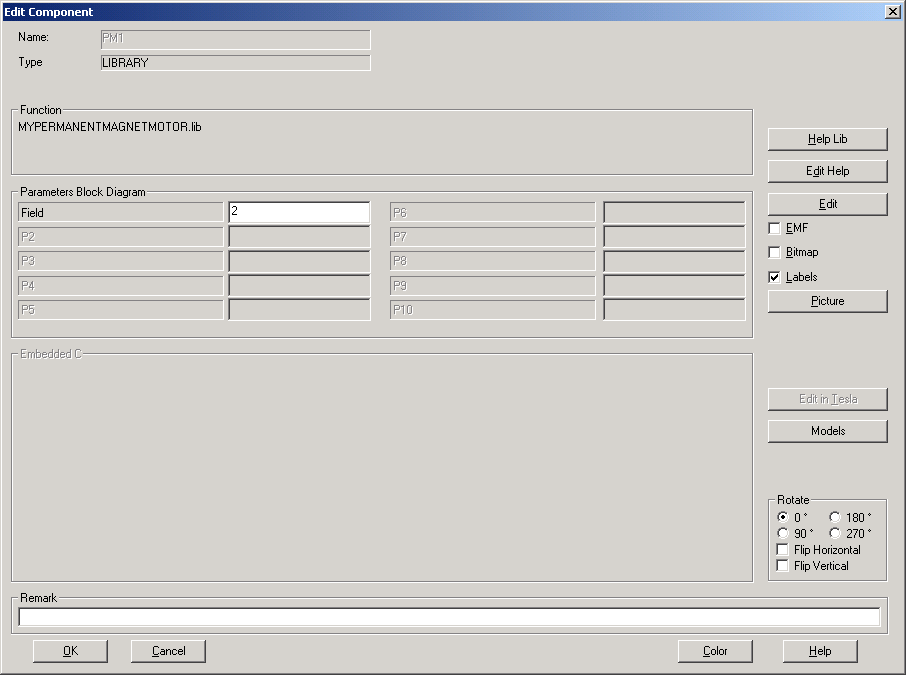Running the simulation again and opening the scope shows the new angualr speed for a Field of 2 Tesla.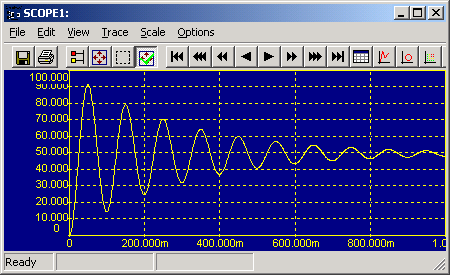Amazing, we doubled our magnetic field, but obtained a lower angular speed of only 50 Rad/s. Well here is the effect that we call field weakening. Of course all our components are linear and there is no saturation. If we set the field to 0.8 Tesla, the angular speed increases to 120 Rad/s.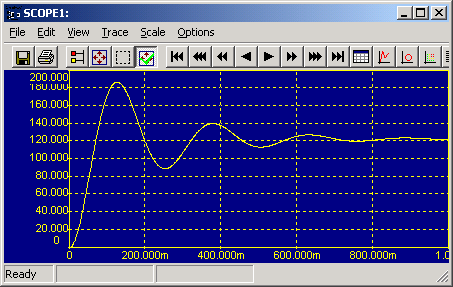The field weakening is only possible if there is no saturation in the machine. Saturation can be modeled by making the inductance dependent on the rotor current. To model the saturation in our PM machine, we open the machine model, by selecting it with the right mouse button and selecting the  button.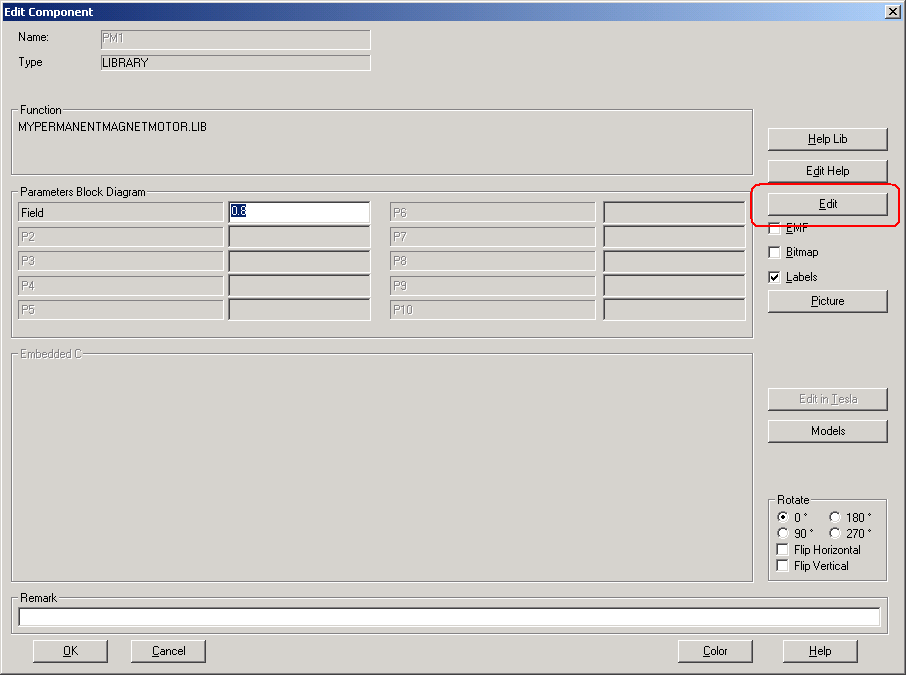Another Caspoc will open with the contents of our PM machine model. Here we can edit the model and we can export the model again in a library block. Since we want to make the inductance dependent on the current through the inductance, we need a block that can change the value of a circuit component during the simulation. This can be achieved with the ChangeE block that we select from Components/Blocks/Miscellaneous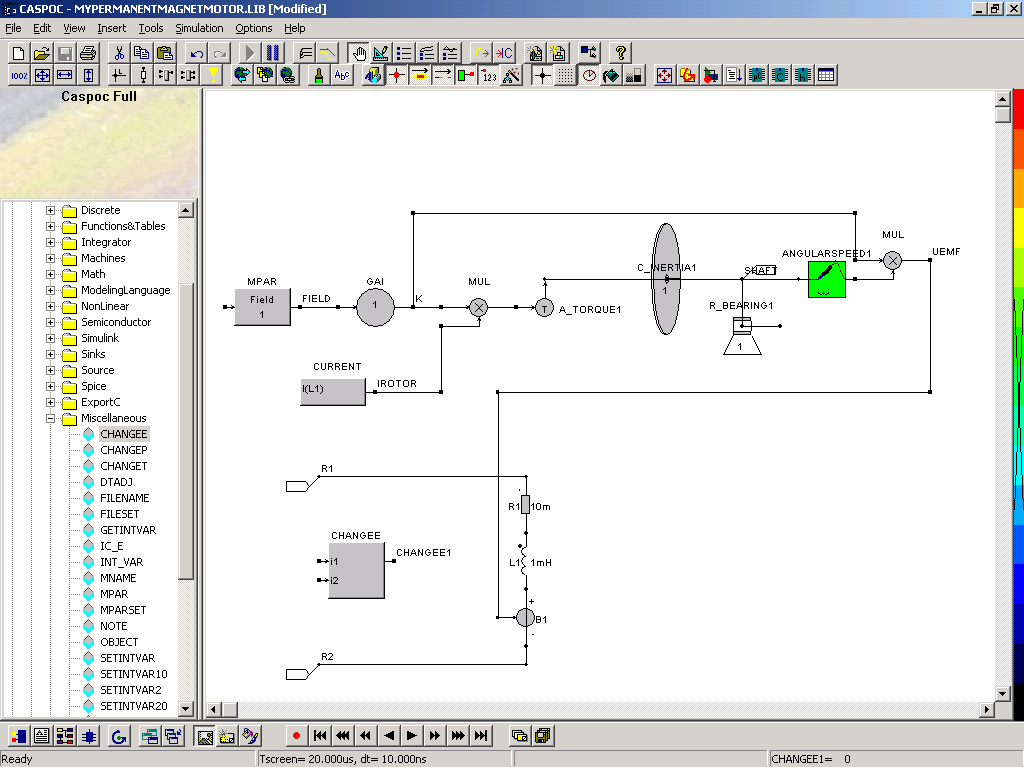A saturation block LSAT selected from Components/Blocks/NonLinear models the independence of the inductance on the inductor current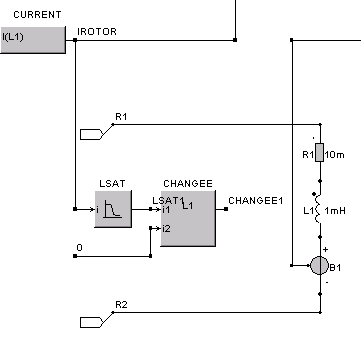Since we want to see the dependency of the inductance on the inductor current, we will export the value of the inductance also as a label from the library block. Export the label LSAT to the top of the library block. Set p1=L0=1mH and p2=i(L=0.5*L0)=2kA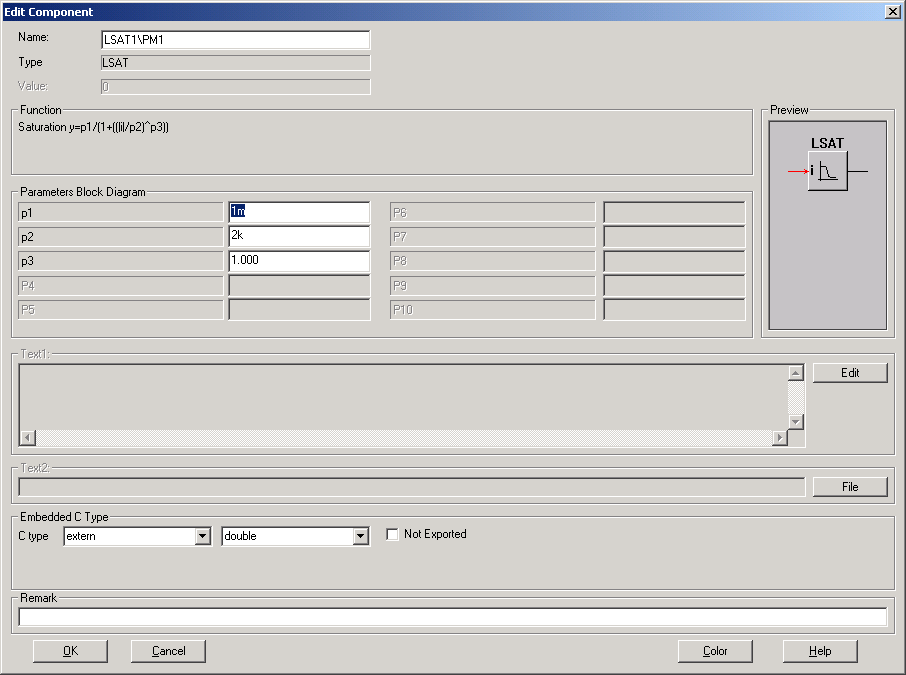Export the library block by selecting Edit/Export Library from the menu and click the [Export] button. Replace the existing file by selecting [Yes] when windows will ask you to overwrite the existing file. Close the Caspoc window in which you edited the PM machine model and you will return to the main schematic. You will notice the extra label that is now visible on the top of the library block. We will use a scope to show the dependence of the inductor on the current. Enlarge the scope to have two inputs and place the second input over he exported label. The first input is connected to the voltage source. Note that a positive measured voltage source current is flowing into the current source. If the current flow out of the source is positive, the measured current is negative.

Run the simulation again open the scope to zoom into the results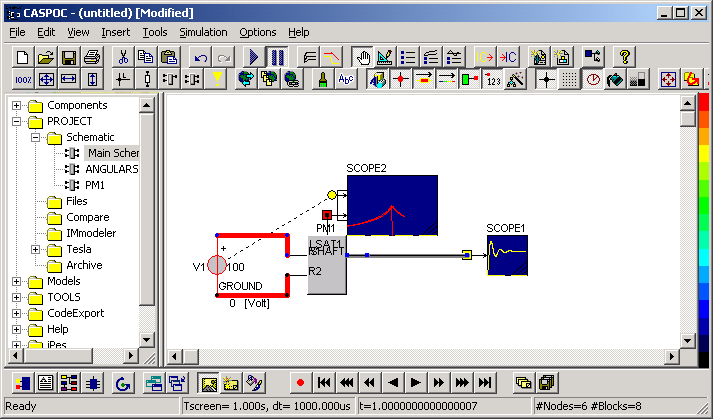When the scope is opened, select the XY view to see the inductor dependency on the current by selecting View/XY Writer from the menu. You can zoom into the results or set the scale by hand. If you set the scale by hand, you first have to disable the automated scaling. Remove the flag at Scale/Auto Resize Scale. Open the scale properties dialog box to set the correct scale directly by selecting Scale/Edit Scale Left and set the following values.If you close the scale properties dialog box, the scope shows the current dependency of the inductor.To see more in detail what is happening inside your machine model, you can view the contents of the model during the simulation. Select Projects/Schematic and select the library block that you would like to see. If a library block is missing from this list, you have to refresh the list by pressing the [F5] function key on your keyboard.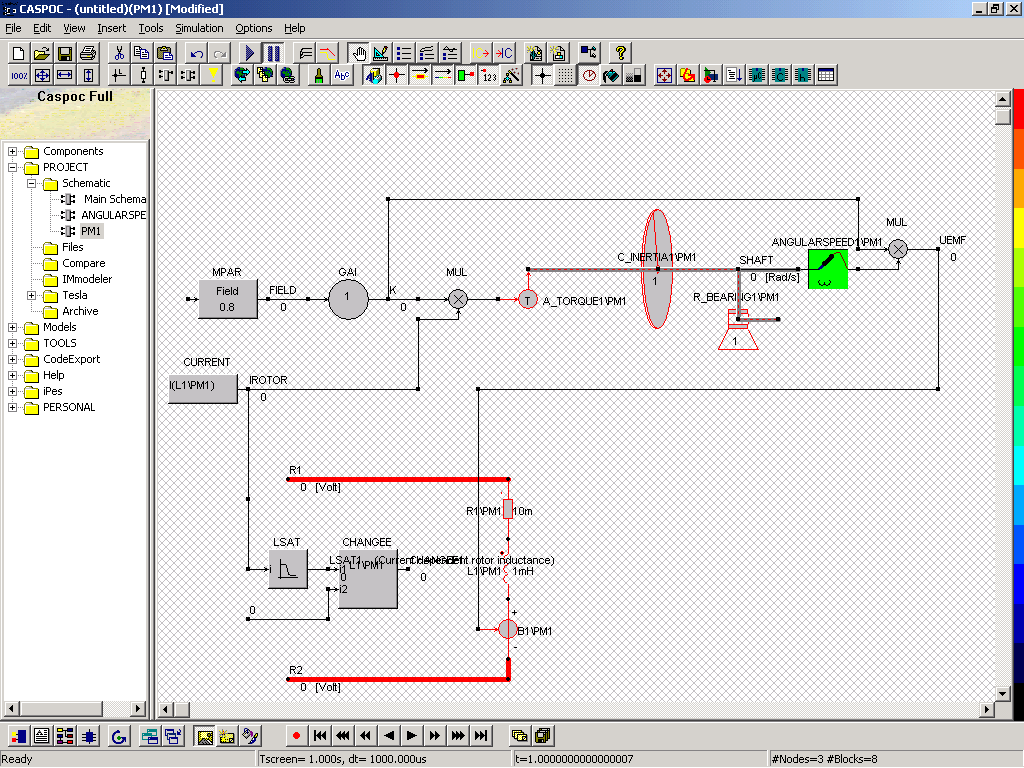Select Main Schematic to see the main schematic, where the library block are used.In the library block we can edit the shematic, add blocks and compoents and add scopes for viewing the simulation results. Note that all editing takes place in the embedded library block. The original library block that is stored on your harddisk remains unaffected. Only the library block that is inserted in your schematic will be changed.

If we would like to see the back-emf we can add a scope and connected its input to the calculated back-emf UEMF.Also the Replay function can be used to view what is happening inside the library block.